Ex 16.3

Chapter 16 Class 11 Probability
Serial order wise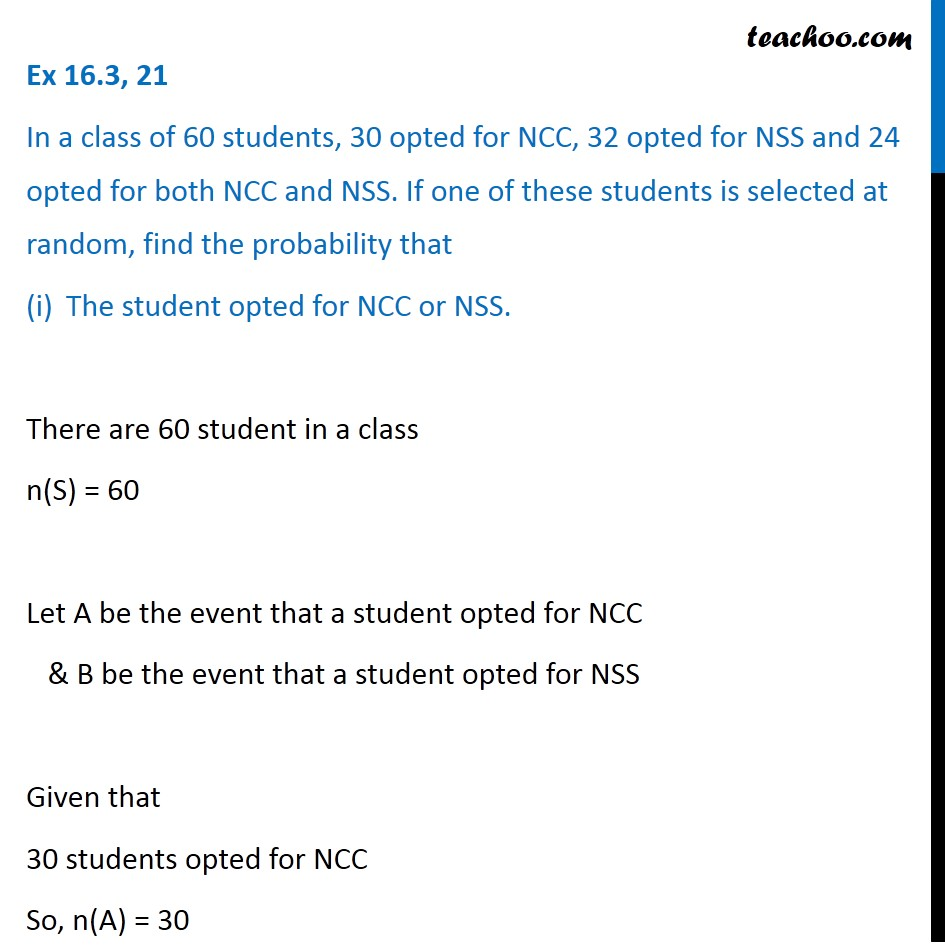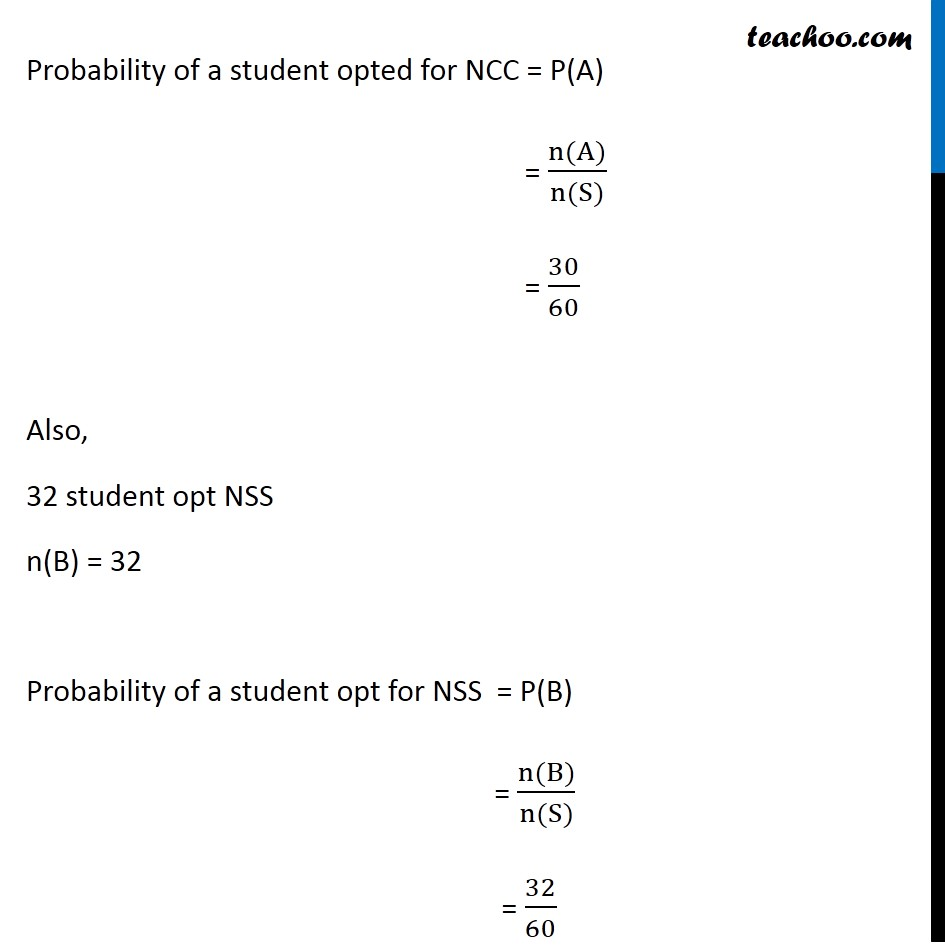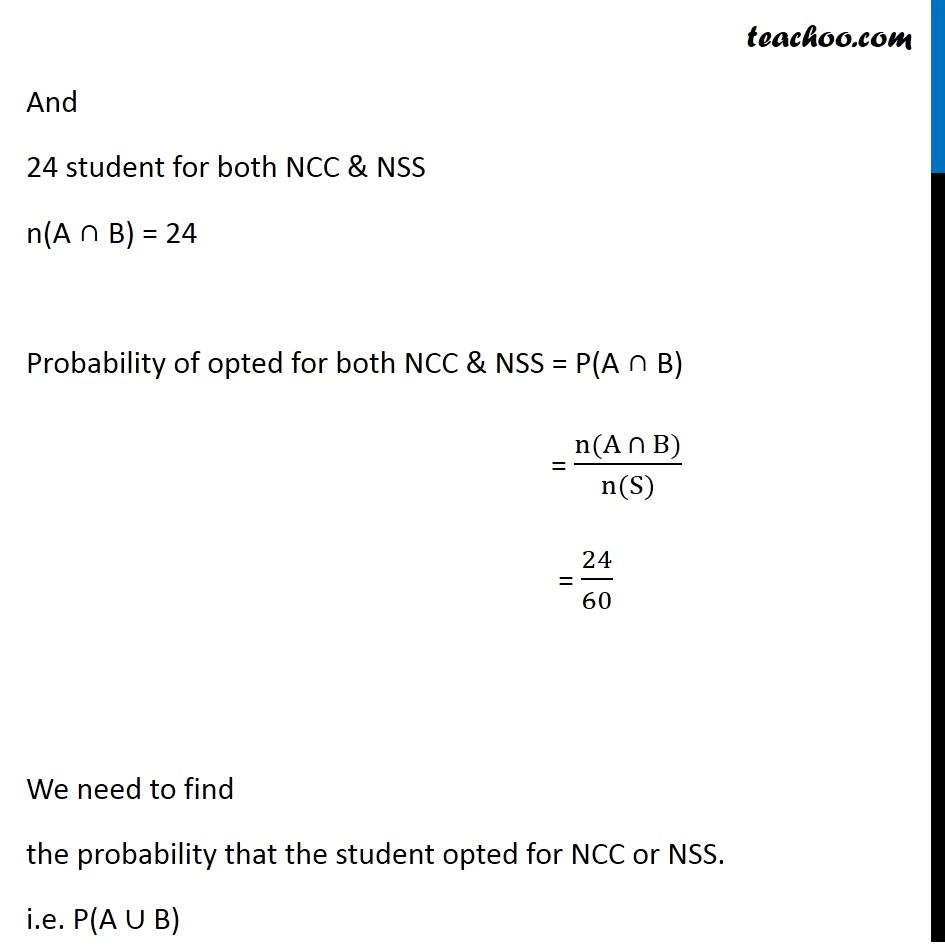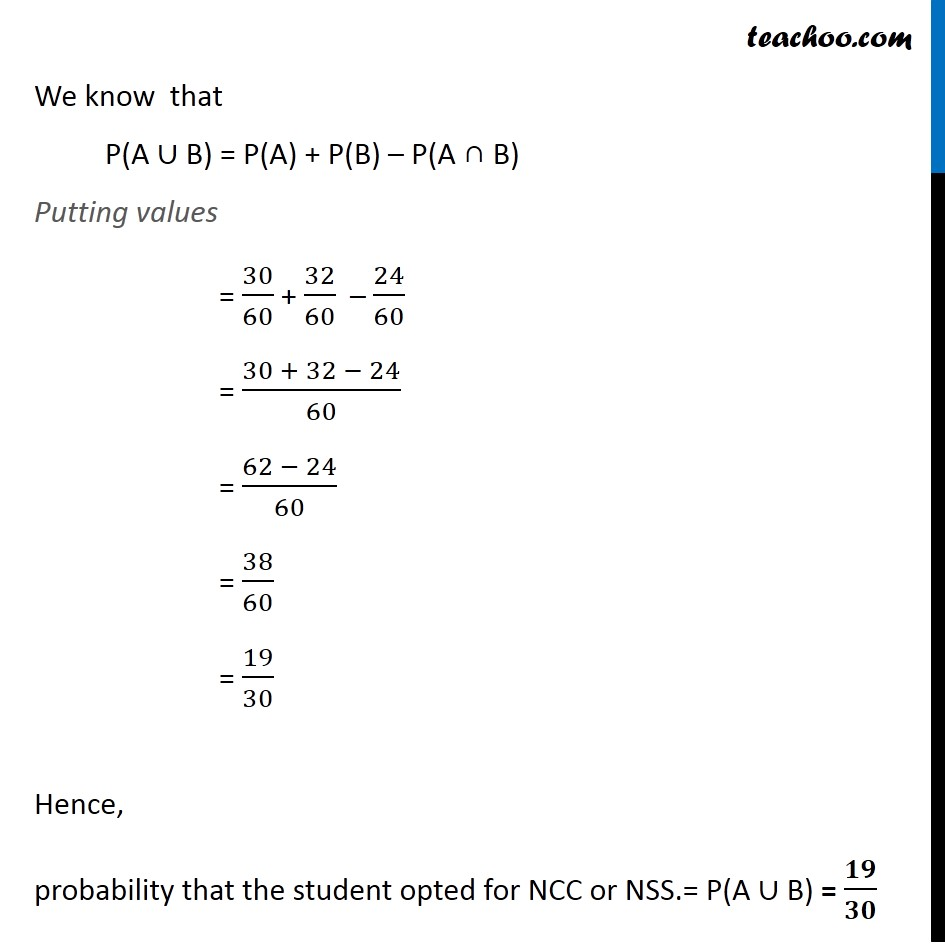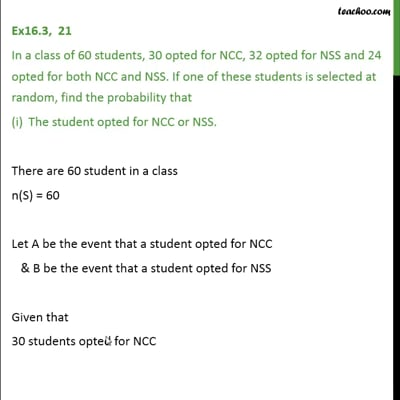This video is only available for Teachoo black users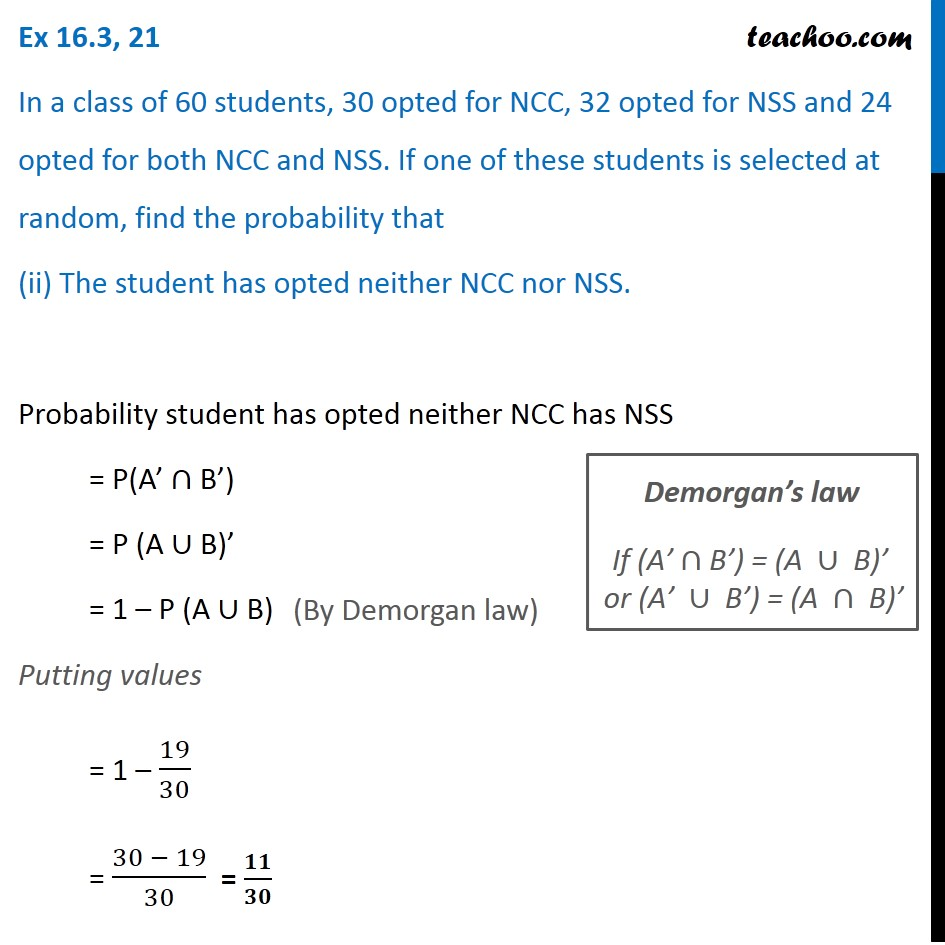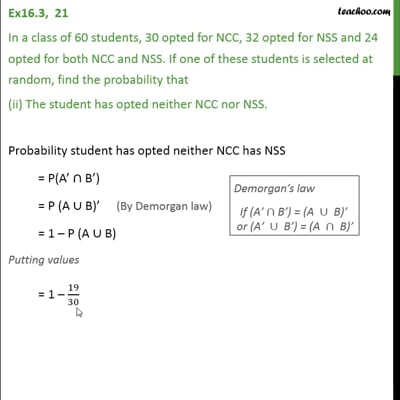This video is only available for Teachoo black users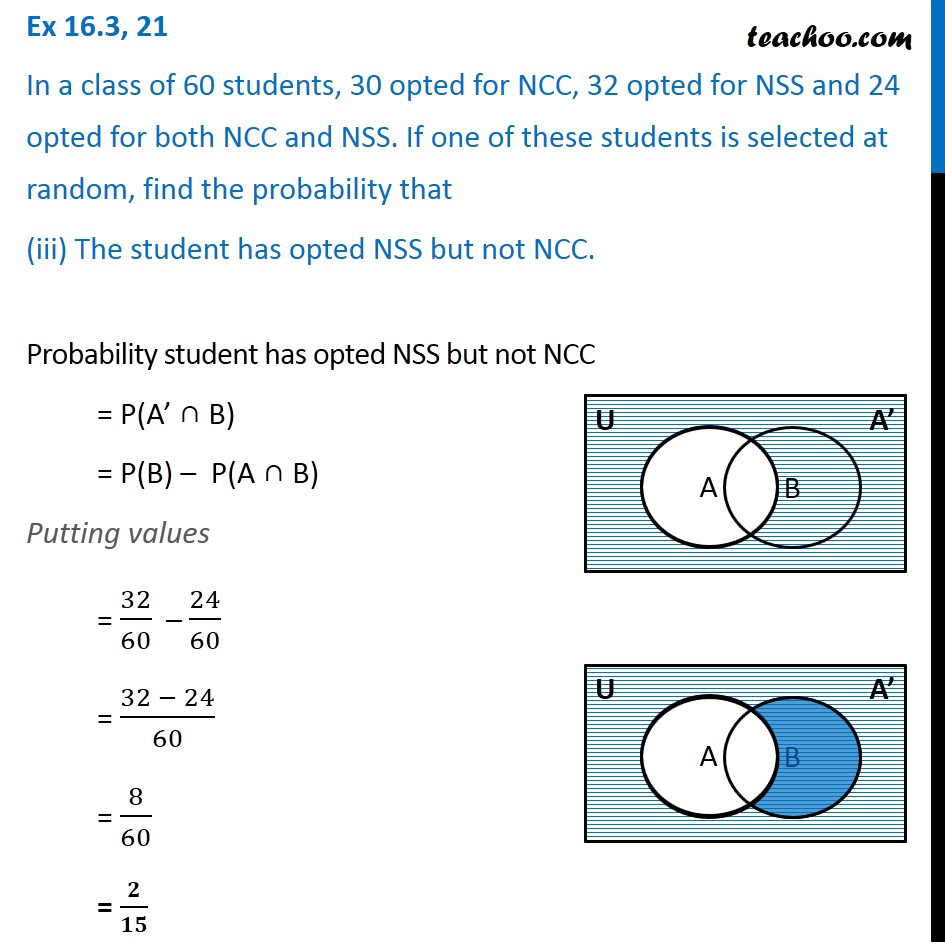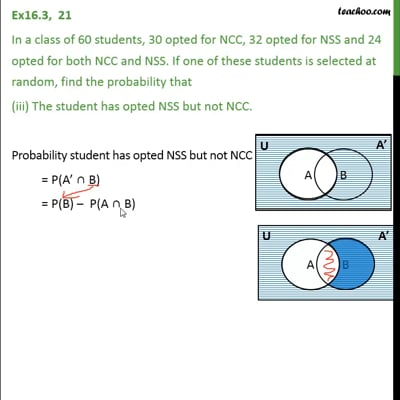This video is only available for Teachoo black users

Solve all your doubts with Teachoo Black (new monthly pack available now!)

### Transcript

Ex 16.3, 21 In a class of 60 students, 30 opted for NCC, 32 opted for NSS and 24 opted for both NCC and NSS. If one of these students is selected at random, find the probability that The student opted for NCC or NSS. There are 60 student in a class n(S) = 60 Let A be the event that a student opted for NCC & B be the event that a student opted for NSS Given that 30 students opted for NCC So, n(A) = 30 Probability of a student opted for NCC = P(A) = (n(A))/(n(S)) = 30/60 Also, 32 student opt NSS n(B) = 32 Probability of a student opt for NSS = P(B) = (n(B))/(n(S)) = 32/60 And 24 student for both NCC & NSS n(A ∩ B) = 24 Probability of opted for both NCC & NSS = P(A ∩ B) = (n(A ∩ B))/(n(S)) = 24/60 We need to find the probability that the student opted for NCC or NSS. i.e. P(A ∪ B) We know that P(A ∪ B) = P(A) + P(B) – P(A ∩ B) Putting values = 30/60 + 32/60 – 24/60 = (30 + 32 − 24)/60 = (62 − 24)/60 = 38/60 = 19/30 Hence, probability that the student opted for NCC or NSS.= P(A ∪ B) = 𝟏𝟗/𝟑𝟎 Ex 16.3, 21 In a class of 60 students, 30 opted for NCC, 32 opted for NSS and 24 opted for both NCC and NSS. If one of these students is selected at random, find the probability that (ii) The student has opted neither NCC nor NSS. Probability student has opted neither NCC has NSS = P(A’ ∩ B’) = P (A ∪ B)’ = 1 – P (A ∪ B) Putting values = 1 – 19/30 = (30 − 19)/30 = 𝟏𝟏/𝟑𝟎 (By Demorgan law) Demorgan’s law █("If (A’" ∩"B’) = (A " ∪" B)’ " @"or (A’ " ∪" B’) = (A " ∩" B)’" ) Ex 16.3, 21 In a class of 60 students, 30 opted for NCC, 32 opted for NSS and 24 opted for both NCC and NSS. If one of these students is selected at random, find the probability that (iii) The student has opted NSS but not NCC. Probability student has opted NSS but not NCC = P(A’ ∩ B) = P(B) – P(A ∩ B) Putting values = 32/60 – 24/60 = (32 − 24)/60 = 8/60 = 𝟐/𝟏𝟓